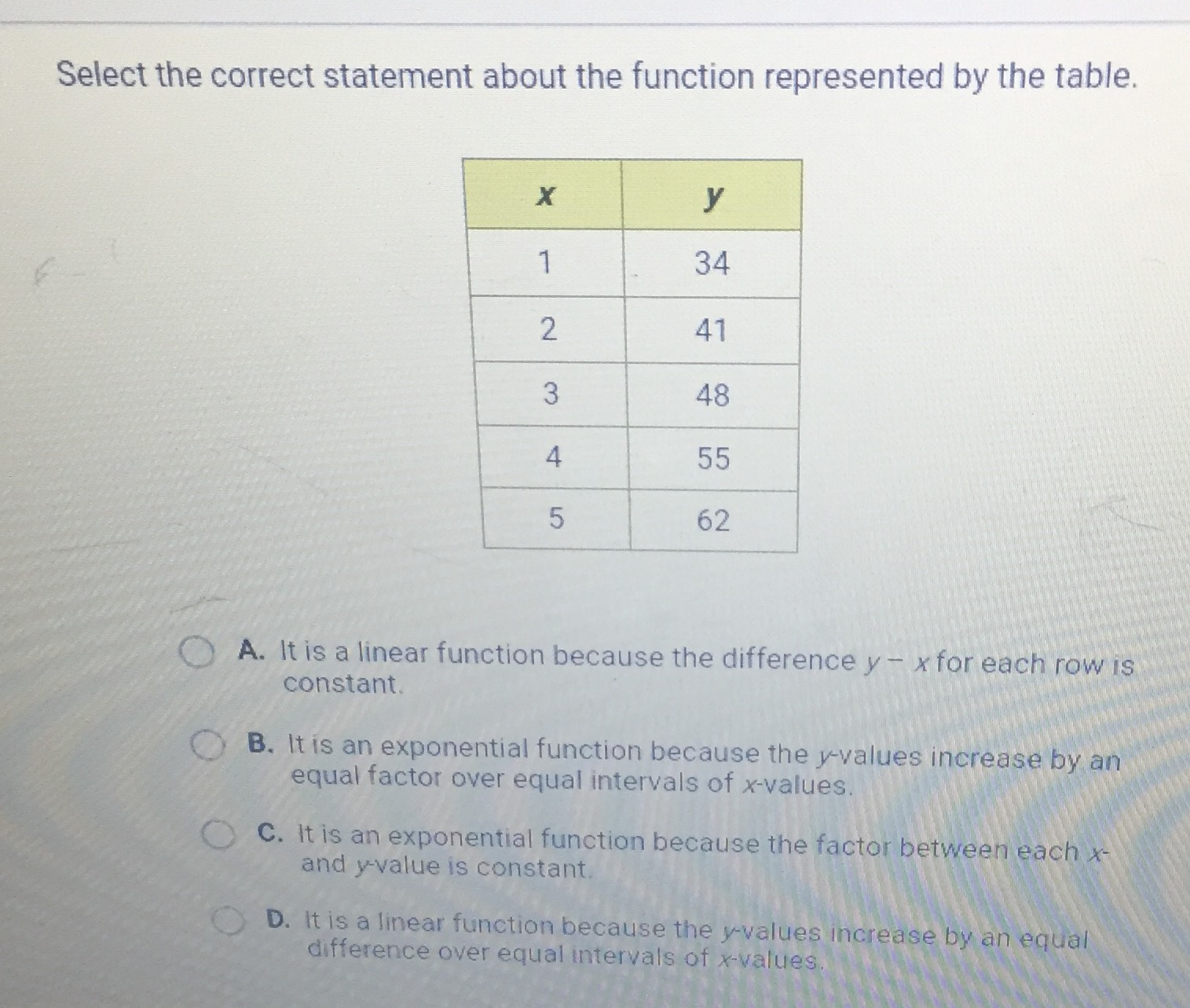### Still have math questions?

Algebra
QuestionSelect the correct statement about the function represented by the table.

A. It is a linear function because the difference $$y - x$$ for each row is constant.

B. It is an exponential function because the $$y$$ -values increase by an equal factor over equal intervals of $$x$$ -values.

C. It is an exponential function because the factor between each $$x$$ - and $$y$$ -value is constant.

D. It is a linear function because the $$y$$ -values increase by an equal difference over equal intervals of $$x$$ -values.